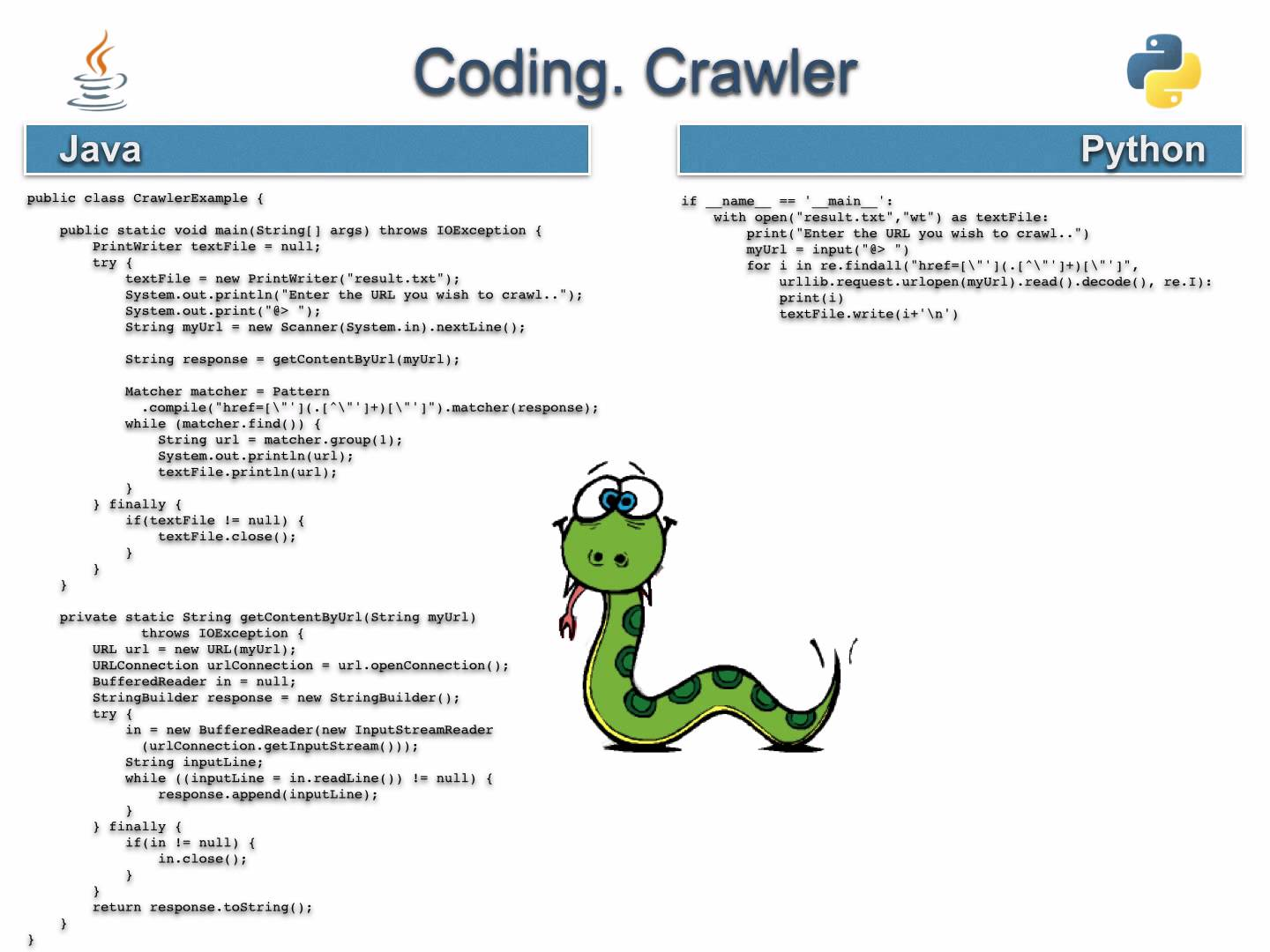# Porting PSLab Libraries – Python to Java

PSLab has existing communication libraries and sensor files in Python which were created during the development of Python Desktop Application.

The initial task and challenge was porting this existing code to Java to be used by the Android App. Since, the python libraries also utilized the object oriented model of programming, porting from Python to Java had the similar code structure and organization.

Common problems faced while porting from Python to Java

• The most common problem is explicitly assigning data types to variables in Java since Python manages data types on its own. However, most of the time the data types are quite evident from the context of their use and understanding the purpose of the code can make the task much simpler.
• Another task was migrating the Python data structures to their corresponding Java counterparts like a List in Python represents an ArrayList in Java, similarly a Dictionary corresponds to a HashMap and so on.
• Some of the sections of the code uses highly efficient libraries like Numpy and Scipy for some mathematical functions. Finding their corresponding Java counterparts in libraries was a challenge. This was partly solved by using Apache Common Math which is a library dedicated for mathematical functions. Some of the functions were directly implemented using this library and for rest of the portions, the code was written after understanding the structure and function of Numpy methods.

While porting the code from Python to Java, some of the steps which we followed:

• Matching corresponding data-structures

The Dictionary in python…

`Gain_scaling = OrderedDict ([('GAIN_TWOTHIRDS', 0.1875), ('GAIN_ONE', 0.125), ('GAIN_TWO', 0.0625), ('GAIN_FOUR', 0.03125), ('GAIN_EIGHT', 0.015625), ('GAIN_SIXTEEN', 0.0078125)])`

…was mapped to corresponding Java HashMap in the manner given below. A point to be noted here is for adding elements to a HashMap can be done only from a method and not at the time of declaration of HashMap.

```private HashMap <String,Double> gainScaling = new HashMap <String,Double>();

gainScaling.put("GAIN_TWOTHIRDS",0.1875);
gainScaling.put("GAIN_ONE",0.125);
gainScaling.put("GAIN_TWO",0.0625);
gainScaling.put("GAIN_FOUR",0.03125);
gainScaling.put("GAIN_EIGHT",0.015625);
gainScaling.put("GAIN_SIXTEEN",0.0078125);
```

Similarly, the List in Python can be  be converted to the corresponding ArrayList in Java.

• Assigning data types and access modifiers to corresponding variables in Java
```POWER_ON = 0x01
gain_choices = [RES_500mLx, RES_1000mLx, RES_4000mLx]
ain_literal_choices = ['500mLx', '1000mLx', '4000mLx']
scaling = [2, 1, .25]```
```private int POWER_ON = 0x01;
public int[] gainChoices = {RES_500mLx,RES_1000mLx,RES_4000mLx};
public String[] gainLiteralChoices = {"500mLx", "1000mLx", "4000mLx"};
public double[] scaling = {2,1,0.25};```

Assigning data types and the corresponding access modifiers can get tricky sometimes. So, understanding the code is essential to know whether a variable in limited to the class or needs to be accessed outside the class, whether a variable is int, short, float or double etc.

• Porting Numpy & Scipy functions to Java using Apache Common Math

For example, this piece of code gives the pitch of acceleration. It uses mathematical functions like arc-tan.

```pitchAcc = np.arctan2(accData, accData) * 180 / np.pi
```

The corresponding version of arc-tan in Apache Common Math is used in Java.

`double pitchAcc = Math.atan2(accelerometerData, accelerometerData) * 180 / pi;`
• Porting by writing the code for Numpy and Scipy functions explicitly

In the code below, rfftfreq is used to calculate the Discrete Fourier Transform(DFT) sample frequencies.

```freqs = self.fftpack.rfftfreq(N, d=(xReal - xReal) / (2 * np.pi))
```

Since, hardly any library in Java supports DFT, the corresponding code for rfftfreq was self-written.

```double[] rfftFrequency(int n, double space){
double[] returnArray = new double[n + 1];
for(int i = 0; i < n + 1; i++){
returnArray[i] =  Math.floor(i / 2) / (n * space);
}
return Arrays.copyOfRange(returnArray, 1, returnArray.length);
}```

After porting of all communication libraries and sensor files are done, the testing of features can also be initiated. Currently, the ongoing development includes porting of the some of the remaining files and working on the the best possible User Interface.